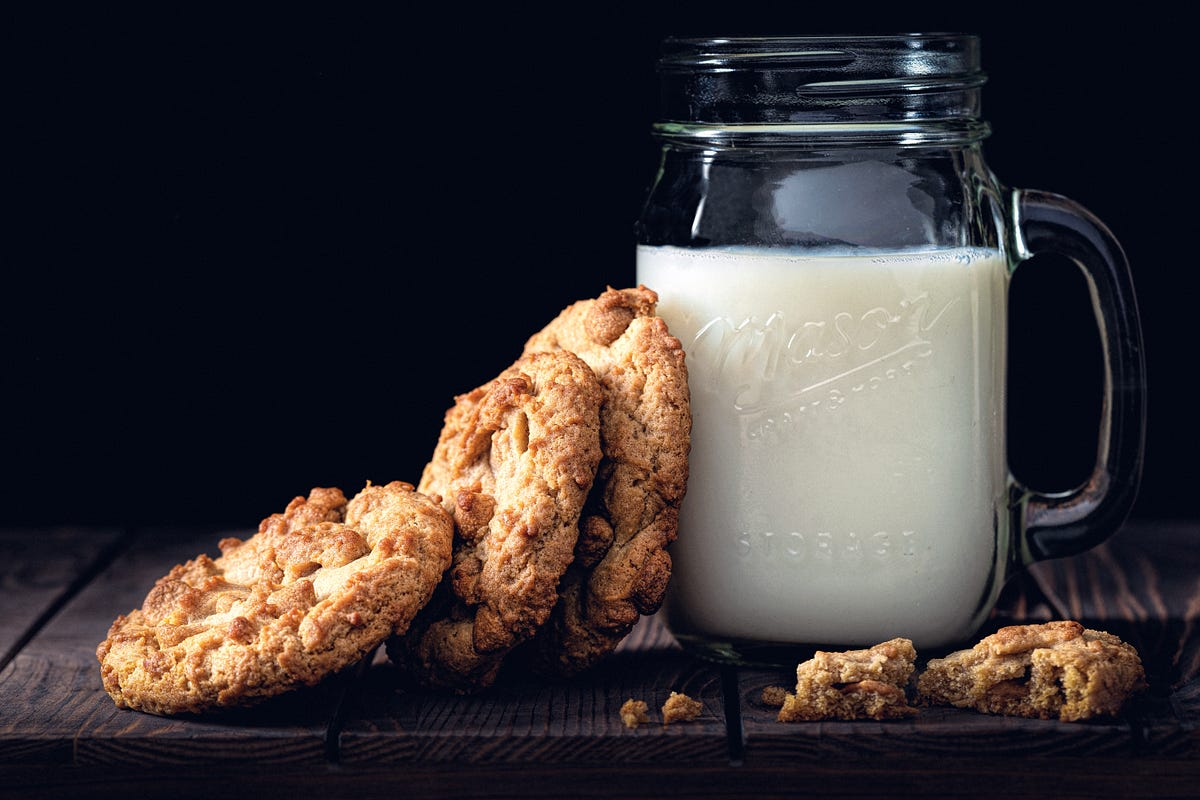1591272360

# Hands-on Time Series Forecasting with Python

Hands-on Time Series Forecasting with Python
Box-Jenkins modeling strategy for building SARIMA model
Time series analysis is the endeavor of extracting meaningful summary and statistical information from data points that are in chronological order.

#python #data-science #forecasting

## Buddha Community1619518440

## top 30 Python Tips and Tricks for Beginners

Welcome to my Blog , In this article, you are going to learn the top 10 python tips and tricks.

### 8) Check The Memory Usage Of An Object.

#python #python hacks tricks #python learning tips #python programming tricks #python tips #python tips and tricks #python tips and tricks advanced #python tips and tricks for beginners #python tips tricks and techniques #python tutorial #tips and tricks in python #tips to learn python #top 30 python tips and tricks for beginners1619510796

## Lambda, Map, Filter functions in python

Welcome to my Blog, In this article, we will learn python lambda function, Map function, and filter function.

Lambda function in python: Lambda is a one line anonymous function and lambda takes any number of arguments but can only have one expression and python lambda syntax is

Syntax: x = lambda arguments : expression

Now i will show you some python lambda function examples:

#python #anonymous function python #filter function in python #lambda #lambda python 3 #map python #python filter #python filter lambda #python lambda #python lambda examples #python map1600660800

## Hands-On Guide To Darts - A Python Tool For Time Series Forecasting

Data collected over a certain period of time is called Time-series data. These data points are usually collected at adjacent intervals and have some correlation with the target. There are certain datasets that contain columns with date, month or days that are important for making predictions like sales datasetsstock price prediction etc. But the problem here is how to use the time-series data and convert them into a format the machine can understand? Python made this process a lot simpler by introducing a package called Darts.

#### Introduction to Darts

For a number of datasets, forecasting the time-series columns plays an important role in the decision making process for the model. Unit8.co developed a library to make the forecasting of time-series easy called darts. The idea behind this was to make darts as simple to use as sklearn for time-series. Darts attempts to smooth the overall process of using time series in machine learning.

#developers corner #darts #machine learning #python #time series #time series forecasting1623226129

## Time Series Analysis Using ARIMA Model With Python

Time series_ is a sequence of time-based data points collected at specific intervals of a given phenomenon that undergoes changes over time. In other words, time series is a sequence taken at consecutive equally spaced points in the time period._

As a example, we can present few time series data sets in different domains such as pollution levels, Birth rates, heart rate monitoring, global temperatures and Consumer Price Index etc. At the processing level, above datasets are tracked, monitored, down sampled, and aggregated over time.

There are different kind of time series analysis techniques in the big data analytical field. Among them few are,

• Autoregression (AR)
• Moving Average (MA)
• Autoregressive Moving Average (ARMA)
• Autoregressive Integrated Moving Average (ARIMA)
• Seasonal Autoregressive Integrated Moving-Average (SARIMA)

ARIMA Model

ARIMA Model is simple and flexible enough to capture relationship we would see in the data and It aims to explain the autocorrelation between the data points using past data. We can decompose the ARIMA model as follow to grab the key elements of it.

• **AR: _Auto regression. _**This is a model that uses the dependent relationship between the data and the lagged data.
• **I:_ Integrated. _**The use of differencing of raw observations (e.g. subtracting an observation from an observation at the previous time step) in order to make the time series stationary.
• **MA: _Moving average. _**A model that uses the relationship between the observations and the residual error from the moving average model applied to lagged observations.

Dataset Explanation

Exploratory Analysis

#python #time-series-analysis #pandas #forecasting #arima #time series analysis using arima model with python1620584520

## Time Series Decomposition in Python – Predictive Hacks

Time series decomposition is a technique that splits a time series into several components, each representing an underlying pattern category, trend, seasonality, and noise. In this tutorial, we will show you how to automatically decompose a time series with Python.

To begin with, lets talk a bit about the components of a time series:

Seasonality: describes the periodic signal in your time series.

Trend: describes whether the time series is decreasing, constant, or increasing over time.

Noise: describes what remains behind the separation of seasonality and trend from the time series. In other words, it’s the variability in the data that cannot be explained by the model.

For this example we will use the Air Passengers Data from Kaggle.

#python #data science #python #statsmodels #time series #time series decomposition